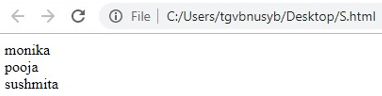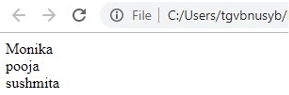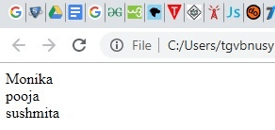# JavaScript Array

0 469

Arrays are used in JavaScript when there is a need to store various values in a single variable. Arrays are also known as objects with extra array-related characteristics, through which one can easily perform actions identical to objects.

Usage of an Array
To store set of numbers or multiple objects with same type.
To store an ordered collection of values.

Syntax:
var student= new Array ("Sushmita", "Pooja", "Monika");

Example:

`<html><body><h2> Arrays in JavaScript</h2><p id="demo"></p><script>var a=["Monika","Pooja","Sushmita"];document.getElementById("demo").innerHTML=a;</script></body></html>`

Output:

Arrays in Javascript

Monika, Pooja, Sushmita

There are three (3) methods to create an Array in JavaScript
1. Literal
2. New keyword
3. Array constructor

1. Literal

Example:

`<script>var student=["monika","pooja","sushmita"];for (j=0;j<student.length;j++){document.write(student[j] + "<br/>");}</script>`

Output:2. New Keyword

Example:

`<script>  var i;  var student = new Array();  student = "Monika";  student = "pooja";  student = "sushmita";    for (j=0;j<student.length;j++){  document.write(student[j] + "<br>");  }  </script>`

Output:3. Array Constructor

`<script>var student=new Array("Monika","pooja","Sushmita");for (j=0;j<student.length;j++){document.write(student[j] + "<br>");}</script>`

Output:Share: